Courses

# Test Level 1: Some Basic Concepts Of Chemistry And Stoichiometry

## 17 Questions MCQ Test Chemistry Class 11 | Test Level 1: Some Basic Concepts Of Chemistry And Stoichiometry

Description
This mock test of Test Level 1: Some Basic Concepts Of Chemistry And Stoichiometry for Class 11 helps you for every Class 11 entrance exam. This contains 17 Multiple Choice Questions for Class 11 Test Level 1: Some Basic Concepts Of Chemistry And Stoichiometry (mcq) to study with solutions a complete question bank. The solved questions answers in this Test Level 1: Some Basic Concepts Of Chemistry And Stoichiometry quiz give you a good mix of easy questions and tough questions. Class 11 students definitely take this Test Level 1: Some Basic Concepts Of Chemistry And Stoichiometry exercise for a better result in the exam. You can find other Test Level 1: Some Basic Concepts Of Chemistry And Stoichiometry extra questions, long questions & short questions for Class 11 on EduRev as well by searching above.
QUESTION: 1

### For the reaction 2x + 3y + 4z → 5w Initially if 1 mole of x, 3 mole of y and 4 mole of z is taken. If 1.25 mole of w is obtained then % yield of this reaction is

Solution:

1 moles of x will give = 5/2 = 2.5 mol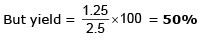QUESTION: 2

Solution:
QUESTION: 3

### 125 ml of 8% w/w NaOH solution (sp. gravity 1) is added to 125 ml of 10% w/v HCl solution. The nature of resultant solution would be ____

Solution: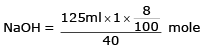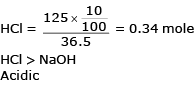QUESTION: 4

Ratio  of masses of H2SO4  and Al2 (SO4)3 is grams each containing 32 grams of S is _____

Solution: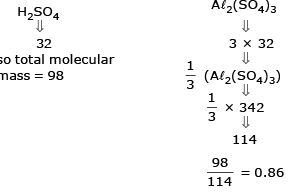QUESTION: 5

The vapour density of a mixture of gas A (Molecular mass = 40) and gas B (Molecular mass = 80) is 25.Then mole % of gas B in the mixture would be

Solution:

Let mole of B = x
V.D = 25   mole of A = 100 x
Mol. mass = 50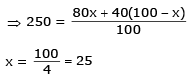QUESTION: 6

For the reaction 2A + 3B + 5C → 3D

Initially if 2 mole of A, 4 mole of B and 6 mole of C is taken, With 25% yield, moles of D which can be produced are _____________.

Solution:

Limiting reactant is A
Ideally with 2 moles of A, D formed = 3 moles But yield = 25%
So, moles of D formed = 3 × 0.25 = 0.75 mol

QUESTION: 7

Two elements X (atomic mass = 75) and Y (atomic mass = 16) combine to give a compound having 75.8% of X. The formula of the compound is:

Solution: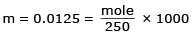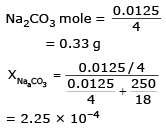QUESTION: 8

Equal volumes of 10% (v/v) of HCl is mixed with 10% (v/v) NaOH solution. If density of pure NaOH is 1.5 times that of pure HCl then the resultant solution be :

Solution:

Both have equal volume = V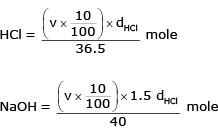NaOH mole > HCl mole
Basic Solution

QUESTION: 9

Similar to the % labelling of oleum, a mixture of H3PO4 and P4O10 is labelled as (100 + x) % where x is the maximum mass of water which can react with P4O10 present in 100 gm mixture of H3PO4 and P4O10. If such a mixture is labelled as 127% Mass of P4O10 is 100 gm of mixture, is

Solution:

P4O10 + 6 H2O → 4H3 PO4
284 gm 108gm 392 gm 108 gm water reacts with P4O10 = 284 gm
27 gm water will react with P4O10 =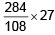= 71 gm

QUESTION: 10

C6H5OH(g) + O2(g) → CO2(g) + H2O(l)

Magnitude of volume change if 30 ml of C6H5OH (g) is burnt with excess amount of oxygen, is

Solution: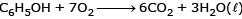30 ml
6 × 30 = 180 ml of CO2 is produced
Volume used initially = 30 + 210 = 240
(for C6H5OH) (for O2)
change in volume = 240 – 180 = 60 ml

QUESTION: 11

Mass of sucrose C12H22O11 produced by mixing 84 gm of carbon, 12 gm of hydrogen and 56 lit. O2 at 1 atm & 273 K according to given reaction, is C(s) + H2(g) + O2(g) → C12H22O11(s)

Solution:

C = 84/12 = 7 mole
H2 = 12 g = 6 mole
O2 = 56/22.4 = 5/2 mole
12C + 11H2 + 11/2 O2 → C12H22O11
L.R. = O2
11/2 mole O2 produce 1 mole sucrose 5/2 mole O2 will for 5/11 mole sucrose mass of sucrose = 5/11 × (mol. mass)
= 5/11 × 342
= 155.45 g

QUESTION: 12

If 50 gm oleum sample rated as 118% is mixed with 18 gm water, then the correct option is

Solution:

118 % ⇒ 100 g sample uses 18 g water
⇒ 50 g sample need 9 g water
(50 g + 18 g water)
⇒ 59 g H2SO4 + 9 g water

QUESTION: 13

In the quantitative determination of nitrogen using Duma’s method, N2 gas liberated from 0.42 gm of a sample of organic compound was collected over water. If the volume of N2 gas collected was 110/11 ml at
total pressure 860 mm Hg at 250 K, % by mass of nitrogen in the organic compound is
[Aq. tension at 250K is 24 mm Hg and R = 0.08 L atm mol–1 K–1]

Solution: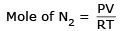P = 860 – 24 = 836 mm Hg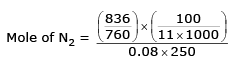= 5 × 10–4 mole mass of N2 = 0.014 g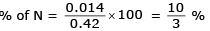QUESTION: 14

The minimum mass of mixture of A2 and B4 required to produce at least 1 kg of each product is:
(Given At. mass of ‘A’ = 10; At mass of ‘B’ = 120) 5A2 + 2B4 → 2AB2  + 4A2B

Solution: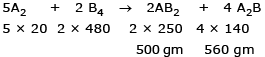Here limiting product is AB2 = 500 gm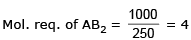So, A2 needed = 10 × 20 = 200 gm
B2 needed = 480 × 4 = 1920 gm
Total mass of mixture = 2120 gm

QUESTION: 15

The % by volume of C4H10 in a gaseous mixture of C4H10, CH4 and CO is 40. When 200 ml of the mixture is burnt in excess of O2. Find volume (in ml) of CO2 produced.

Solution:

C4H10 = 80 ml
CH4 = xml  CO = y ml
x + y = 120 ml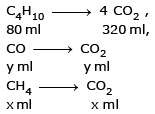total CO2 volume
= 320 + x + y ml
= 320 + 120
= 440 ml

QUESTION: 16

74 gm of sample on complete combustion gives 132 gm CO2 and 54 gm of H2O. The molecular formula of the compound may be

Solution: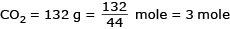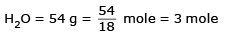⇒ C atoms = 3 mole
H atoms = 6 mole

QUESTION: 17

Density of a gas relative to air is 1.17. Find the mol. mass of the gas. [Mair = 29 g/mol]

Solution: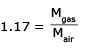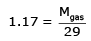M gas = 29 × 1.17 = 33.9